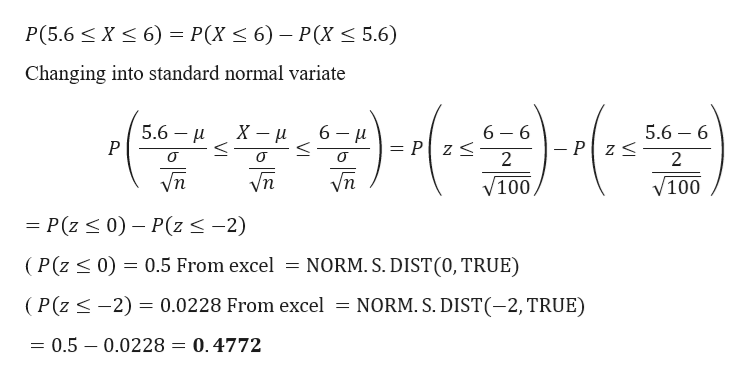# 5. Answer the following in details and steps. Thanks.a. The length of time it takes to fill an order at a ABC sandwich shop is normally distributed with a mean of 6 minutes and a standard deviation of 2 minute. What is the probability that the average waiting time for a random sample of 100 customers is between 5.6 and 6 minutes?  b. The length of time it takes to fill an order at a DEF sandwich shop is normally distributed with a mean of 5 minutes and a standard deviation of 2 minute. Suppose the probability that the average waiting time for a random sample of customers between 4.75 and 5 minutes is 0.3413. What is the number of customers in the sample? c. The length of time it takes to fill an order at a XYZ sandwich shop is normally distributed with a mean of 7 minutes. Suppose the probability that the average waiting time for a random sample of 36 customers between 6.8 and 7.2 minutes is 0.383. What is the standard deviation of the waiting time?

Question
2 views

5. Answer the following in details and steps. Thanks.

a. The length of time it takes to fill an order at a ABC sandwich shop is normally distributed with a mean of 6 minutes and a standard deviation of 2 minute. What is the probability that the average waiting time for a random sample of 100 customers is between 5.6 and 6 minutes?

b. The length of time it takes to fill an order at a DEF sandwich shop is normally distributed with a mean of 5 minutes and a standard deviation of 2 minute. Suppose the probability that the average waiting time for a random sample of customers between 4.75 and 5 minutes is 0.3413. What is the number of customers in the sample?

c. The length of time it takes to fill an order at a XYZ sandwich shop is normally distributed with a mean of 7 minutes. Suppose the probability that the average waiting time for a random sample of 36 customers between 6.8 and 7.2 minutes is 0.383. What is the standard deviation of the waiting time?

check_circle

Step 1

Hello there! there are more than one question. According to our policies we cannot answer more than one question. So please find the solution for first question. If you have doubts in remaining part of the question kindly make a n...help_outlineImage TranscriptioncloseP(5.6 < X < 6) = P(X < 6) – P(X < 5.6) Changing into standard normal variate 5.6 – 6 5.6 — и 6 - u 6 – 6 = P z < Vn vn yn V100 V100 = P(z < 0) – P(z<-2) = NORM. S. DIST(0, TRUE) (P(z < 0) = 0.5 From excel (P(z <-2) = 0.0228 From excel = NORM. S. DIST(-2, TRUE) 0.5 – 0.0228 = 0.4772 fullscreen

### Want to see the full answer?

See Solution

#### Want to see this answer and more?

Solutions are written by subject experts who are available 24/7. Questions are typically answered within 1 hour.*

See Solution
*Response times may vary by subject and question.
Tagged in

### Other